# Class 9 Maths notes for Lines And Angles

Welcome to Class 9 Maths chapter 6 Lines and Angles notes.The topics in this page are types of angle,Complementart,supplimentary angles, Sum of Angle of the triangle along with examples, and questions and answers .This is according to CBSE and the NCERT textbook. If you like the study material, feel free to share the link as much as possible.

We have already studied point,line,line segment in previous chapter.
a. Point: It is denoted by a single dot on the paper and it is represented by English alphabet. It has no length ,breadth and thickness
b. Line: A line is straight and has no curves. It extends in both the direction and has no end point. We have infinite lines through a point while we will have only one line through two points
c. Line Segment:If we take a portion of a line ,then it is called line segment and it has length and two end points. It can be measured
d. Ray: If a line has one end point and it can extent in other direction,then it is called a ray
e. Congruent segments: If two line segments are equal then they are called congruent
Euclid Geometry
Now we will extend our knowledge to angles in this chapter

Table of Content

## What is angle

An angle is formed by two rays with a common endpoint. The Common end point is known as the vertex of the angle and the rays as the sides, sometimes as the legs and sometimes the arms of the angle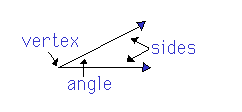We denote the angle by symbol $\angle$
If A is the Vertex,then angle could be represented as $\angle A$

## Types of Angle

As the angle size is increased,it is termed with different name.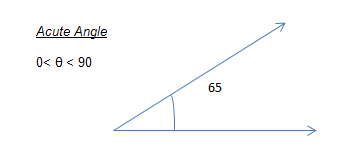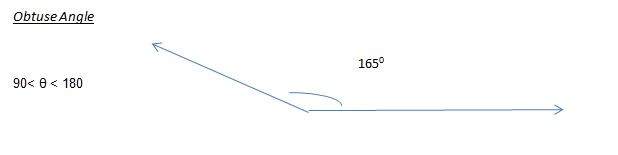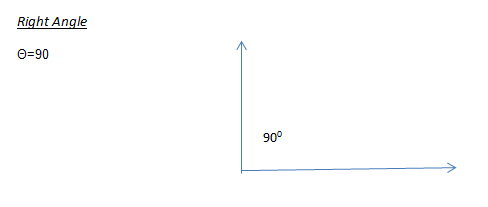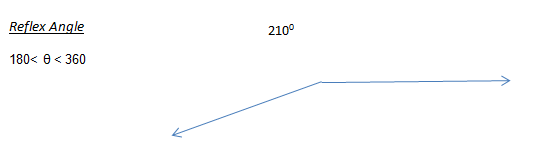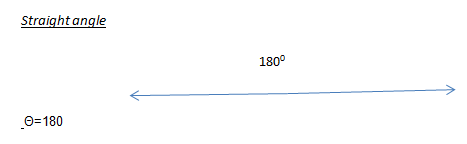They both are unit of measurement of angles
Radian: A unit of measure for angles. One radian is the angle made at the centre of a circle by an arc whose length is equal to the radius of the circle.
Degree: If a rotation from the initial side to terminal side is (1/360) of a revolution, the angle is said to have a measure of one degree, written as 1°. A degree is divided into 60 minutes, and a minute is divided into 60 seconds . One sixtieth of a degree is called a minute, written as 1", and one sixtieth of a minute is called a second, written as 1'.
Thus, 1° = 60', 1' = 60"
 Degree 30° 45° 60° 90° 120° 180° 360° Radian π/6 π/4 π/3 π/2 2π/3 π 2π

## Congruence Angle

Two angles are said to be congruent if they are having same measure
$\angle A=30^0$
$\angle B=30^0$
then
$\angle A=\angle B$
They are congruent

• Two angles are called adjacent angles if they share the same vertex,they have a common arm. The second arm of the one angle is one side and second arm of other angle is on the other side
• Example given below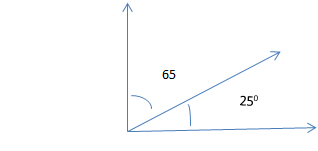## Complimentary Angles

• Two angles , the sum of whose measures is $90^0$ is called Complimentary Angles.Each of these Complimentary Angles are called the complement of each other
• Example given belowHere the sum of angles =$90^0$

## Supplementary Angles

• Two angles , the sum of whose measures is $180^0$ is called Supplementary Angles.Each of these Supplementary Angles are called the supplement of each other
• Example given below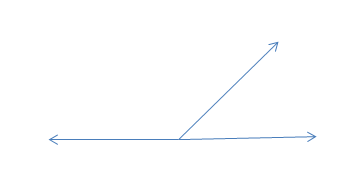Here the sum of angles =$180^0$

## Linear Pair Axioms

• If a ray stands on a line, then the sum of the adjacent angles so formed is $180^0$
• And If the sum of the adjacent angles is $180^0$,then the non common arms of the angles form a line
•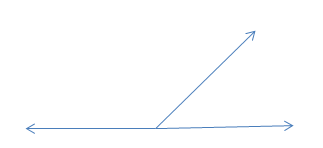Theorem Based on Linear Pair Axiom
The sum of all the angles around a point is $360^0$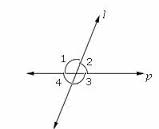$\angle 1 + \angle 2 + \angle 3 + \angle 4 =360^0$

## Vertically Opposite angles

If two lines intersect with each other, then vertically opposite angles are equal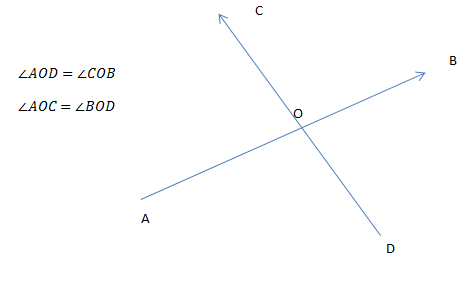## Transversal across the parallel Lines

If the transversal intersect two parallel lines as shown in below figure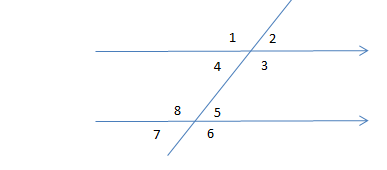Important Take away from the figure
1. We can see following angles as depicted in the figure above
$\angle 1,\angle 2,\angle 3,\angle 4$ on the first parallel line
and $\angle 5,\angle 6,\angle 7,\angle 8$ on the second parallel line
2. The angles 1,2,6,7 are called exterior angles while the angles 4,3,5,8 are called interior angles
exterior angles = $\angle 1, \angle 2, \angle 6 , \angle 7$
interior angles = $\angle 4, \angle 3, \angle 5 , \angle 8$
3. Corresponding Angles The angles on the same side of the Transversal are known as Corresponding angles
And Corresponding Angles axiom states that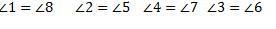4. Each pair of alternate interior angles are equal. This is a theorem which can be obtained from the above axioms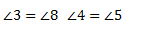5. Each pair of interior angles on the same side of the transversal is supplementary.This is a theorem which can be obtained from the above axioms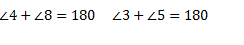## Converse of Transversal across the parallel Lines

If a transversal intersect two lines such that either
1. Any one pair of corresponding angles are equal
2. any one pair of alternate interior angles are equal
3. any one pair of interior angles on the same side of the transversal is supplementary
Then the two lines are parallel

## Parallel lines Note

Lines which are parallel to a given line are parallel with each other

## Angle sum property of Triangles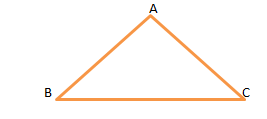• The sum of the angles of the triangle is $180^0$
• if the side of the triangle is produced ,the exterior angle formed is equal to the sum of the opposite interior angle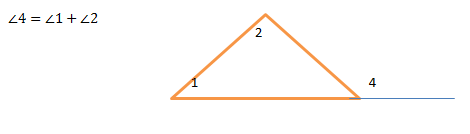## Solved examples

Question 1
The angles of a triangle are in the ratio 5 : 3 : 7. The triangle is :

(a) an acute angled triangle
(b) an obtuse angled triangle
(c) a right triangle
(d) an isosceles triangle

Solution
(a) an acute angled triangle
Let the angles be 5x, 3x and 7x. Using angle sum property, $5x + 3x + 7x = 180^0$
$15x = 180^0$
$x = 12^0$
Hence angles $60^0, 36^0, 84^0$ .SO it is an acute angle triangle.

Question 2
An exterior angle of a triangle is $130^0$ and its two interior opposite angles are equal. Each of the interior angle is equal to:
(a) $45^0$
(b) $65^0$
(c) $75^0$
(d) $35^0$
Solution
Let x be the two angles equal
then $2x + 50=180$
$x=65$

Question 3
True and false statement
(a) A triangle can have two right angles
(b) A triangle can have two obtuse angles
(c) A triangle can have two acute angles
(d) A triangle can have all angles less than $60^0$
(e) A triangle can have all angles more than $60^0$
(f) A triangle can have all angles equal to $60^0$
g) The two acute angles in every right triangle are complementary.

Solution
(a) False. Since the sum of three angles of a triangle is $180^0$. Sum of two right angles is 180, such triangle is not possible.
(b) True Because the sum of two obtuse angles will become greater than $180^0$. Such a triangle is not feasible.
(c) True a triangle can have two acute angles. Since the sum of two acute angle is less than $180^0$, the third angle will have the remaining value.
(d) False Since sum of all angles less than $60^0$ is still less than $180^0$. Such a triangle is not possible.
(e) False Since sum of angles (each angle is greater than $60^0$) exceed $180^0$, such a triangle is not possible.
(f) True Sum of angles (each = $60^0$) is exactly equal to $180^0$, such a triangle is possible. It makes an equilateral triangle.
g) True
Crossword for Line and Angle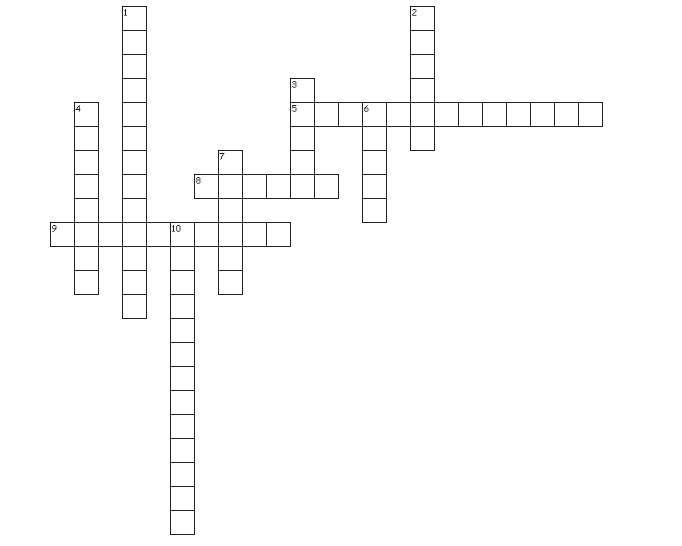Across
5. The angles on the same side of the Transversal are known as
8. Unit of angle
9. If two lines intersect with each other, then _______ opposite angles are equal
Down
1. Angles whose sum is $180^0$
2. Angle( $90^0 < \theta < 180^0$ )
3. Angle( $0^0 < \theta < 90^0$ )
4. Angles which share one side
6. Angle ($\theta=90^0$
7. Angle ($180^0 < \theta < 360^0$ )
10. Angles whose sum is $90^0$

## Quiz Time

Question 1Find the value of x for which line l and m are parallel ?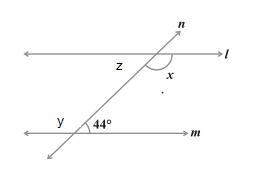A. $136^0$
B. $44^0$
C. $46^0$
D. none of the above
Question 2Find the value of z in the above questions
A. $136^0$
B. $44^0$
C. $46^0$
D. none of the above
Question 3 Find the value of y
A. $136^0$
B. $44^0$
C. $46^0$
D. none of the above
Question 4 The angles of a triangle are in the ratio 2 : 3 : 4. Find the angles of the triangle
A. $80^0,120^0,160^0$
B. $20^0,30^0,40^0$
C. $40^0,60^0,80^0$
D. None of the above
Question 5 The lines AB, CD and EF are three lines concurrent at O as shown below in figure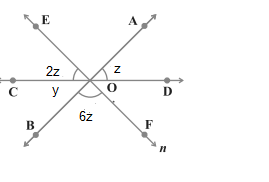Find the value of $\angle EOC$> A. $12^0$
B. $30^0$
C. $80^0$
D. $40^0$
Question 6 In the above question, find the angle $\angle COB$ ?
A. $12^0$
B. $30^0$
C. $20^0$
D. $40^0$

## Summary

Here is Lines and angles class 9 notes summary
• If a ray stands on a line, then the sum of the two adjacent angles so formed is 180°. This is called linear pair axiom
• If two lines intersect each other, then the vertically opposite angles are equal
• The sum of the three angles of a triangle is 180°
• If the transversal intersect two parallel lines, then each pair of corresponding and alternate angles are equal and each pair of interior angles on the same side of the transversal is supplementary